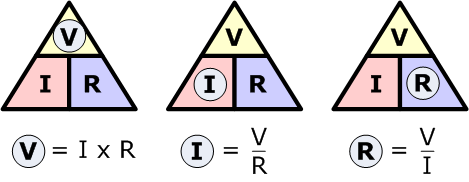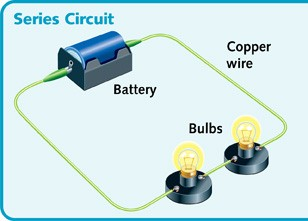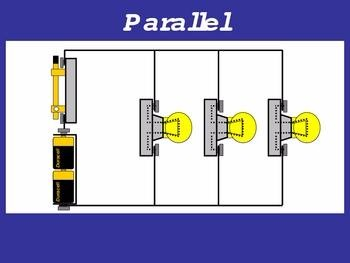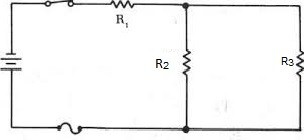Click to Chat

1800-1023-196

+91-120-4616500

CART 0

• 0

MY CART (5)

Use Coupon: CART20 and get 20% off on all online Study Material

ITEM
DETAILS
MRP
DISCOUNT
FINAL PRICE
Total Price: Rs.

There are no items in this cart.
Continue Shopping• Complete Physics Course - Class 11
• OFFERED PRICE: Rs. 2,968
• View Details

```Combination of Resistors – Series and Parallel

Table of Content

Introduction to Combination of Resistors – Series and Parallel

Circuit Parameters

Ohm’s Law

Series and Parallel Connections

Combination of resistors in series connection

Combination of resistors in Parallel Connection

Combination of series and parallel resistors

Summary

Introduction to Combination of Resistors – Series and Parallel

Electrical current is the flow of charged particles. The flow of charges will be constant in current electricity. Electric current flows from higher electric potential to lower electric potential. For the current to flow, it requires a circuit which is closed loop of a conducting material. The circuit consists of wires which are connected end to end and the electrons flow in same direction.

A circuit has conductors (wire), switch, load and a power source. The circuit starts and stops at the same point. Usually copper wires are used as conductors without insulation. It is through the conductor the current flows. A switch is used to open or close the circuit. When the switch is closed current flows through the circuit and when the switch is open, it breaks the circuit and no current flows through it. A cell can be the power source. If we put more than one cell it becomes a battery.

The load which is also known as Resistor uses the electrical energy and converts it into some other form of energy. It can be a light bulb or anything else. If there is no load in the circuit then it will be a short circuit.A circuit

Circuit Parameters

Electric Current is measured in amperes (amps) by an ammeter which will be connected in series with other components in a circuit. Current, I = Q / t, where Q is the charge in coulombs and t is the time in seconds. Andre Marie Ampere found that two parallel wires attract each other when the electric current flows in one direction. Also, two parallel wires repels one another when the electric current flows in opposite directions. As a result of his discoveries in this area, the unit of current is derived from his name which is called as ‘amp’. One amp is equal to one coulomb of charge per second of time.

Voltage is defined as the electrical potential difference between two points in a circuit. The unit of voltage is volts. The unit is derived from the name Alessandro Volta. Cells or batteries provide the voltage or the potential difference which is required.

Resistance resists the flow of current. It is the measure of an objects ability to hold off the flow of electrons. The resistance will be low in a conductor and high in an insulator. It is measured in ohms. The unit ohm is named after the scientist Georg Simon ohm who formulated the ohm’s law.

Resistors are used to control the flow of electric current in a circuit. It converts electrical energy into heat and light. A resistor is a passive component as they consume power but will not generate power. They are usually made up of metal, carbon or with metal oxide film. Resistors are used for limiting the current and to protect the semiconductor devices like LED. It is also used to limit the frequency response in a filter circuit.

Ohm’s Law

Georg Simon Ohm showed the relationship between voltage, current and resistance and formulated the ohm’s law. This law is the basis of electricity.

The law states that V = I R, where voltage V is in volts, Current I in amps and resistance R in ohms.

Thus I = V / R and R = V / I.

For more clarity, electricity can be related to water. Thus the voltage in a circuit whose unit is volts is identical to the pressure of water which is flowing in a pipe. The current in a circuit, where the unit is amps, is equivalent to the water flowing through a pipe. The resistance in the circuit, whose unit is ohms is same as that of the friction and size of the pipe which restricts the flow the water.Ohm’s Law

Series and Parallel Connections

There are mainly two types of circuits, Series and Parallel. Both series and parallel circuits consists of more than one load. Resistors can be connected both in series, parallel or a combination of both.

In series circuit electrons travel only in one path. Here the current will be the same which passes through each resistor. The voltage across resistor in a series connection will be different. In series connection if one resistor is broken or any fault occurs, the entire circuit is turned off. Series circuits do not overheat easily. The design of series circuit is simple compared to parallel circuits.

Some Christmas tree lights can be in series circuits. If one bulb goes off the entire string goes out. The fuse or the breakers will be connected in series to protect the whole wiring from over current. It can be used as a voltage divider. Batteries in a remote are connected in series.Series circuit

In parallel circuit electrons travel through many branches in it. In this case the voltage remains the same across each resistors in the circuit. Here the current in the circuit is divided among each branches and finally recombines when the branches meet at a common point. A parallel circuit can be formed in many ways, which means resistors can be arranged in different forms. It can be used as a current divider.

In most cases the circuits are connected in parallel. This is because if one resistor is broken or damaged, it won’t turn off the entire system. But due to this effect, it is difficult to detect the failure if anything goes wrong in the circuit and thus it can be dangerous at certain times. It is easy to connect or disconnect a new resistor or other component without affecting the other elements in the parallel circuit. But it uses a lot of wires and hence becomes complex. Mostly in buildings and houses we use parallel connection.Parallel circuit

Combination of resistors in series connection

Consider three resistors R1, R2, R3 which are connected in series. Here the charge first flows through R1 and enters R2 and finally reaches R3.Combination of three resistors in series

By ohm’s law, the potential difference across R1 =V1= I R1

The potential difference across R2 = V2 = I R2.

The potential difference across R3 =V3 = I R3.

Thus, the potential difference V across this series connection of resistors

V = V1 + V2 + V3

= I R1 + I R2 + I R3

= I (R1+ R2+ R3)

Thus, in case of series connection, the equivalent resistance, Req = V/I = (R1+ R2+ R3).

For n number of resistors connected in series, the equivalent resistance Req = R1+ R2+ R3…………………Rn.

Equivalent resistance is the total resistance of the circuit. It is the single value of resistance which may replace the number of the resistors in the circuit without changing current and voltage in the network. Thus, in a series connection, the total resistance of a circuit is determined by adding the resistance of each individual resistors.

For Example, consider a series circuit which consists of three resistors with resistance 5Ω, 10Ω, 5Ω respectively with a 15v battery.

So, the total resistance R = R1 + R2 + R3 = 5 + 10 + 5 = 20Ω

We know that V= I R.

Current I = V / R = 15/20 = 0.75A. Though the current in the series network is the same, the voltage drop across each resistor is different. Each resistor with different resistance provide different voltage drop and we can find the total voltage by ohm’s law, V = I R. Let’s take the previous example. The voltage mentioned here is 15v. We can verify that by calculating in this way. V = I R1 + I R2 + I R3 = 0.75 (5 +10 + 5) = 15v. This is the exact measurement of voltage we provided in this example. It is found that the resistor with more resistance has more voltage drop.

Combination of resistors in Parallel Connection

Consider three resistors R1, R2, R3 which are connected in parallel. The charge splits into three and flows through R1, R2 and R3.Combination of three resistors in parallel

Current I = I1 + I2 + I3.

The potential difference applied to R1 = V = I1 R1

The potential difference across R2 = V = I2 R2

The potential difference across R3 = V = I3 R3

Thus I = I1 + I2 + I3

= V/ R1 + V/ R2 +V/ R3

=V (1/ R1 +1/ R2 +1/ R3)

If this parallel combination is replaced by an equivalent resistance, Req

Then I = V/ Req

1/ Req = 1/ R1 +1/ R2 +1/ R3

Thus, for n number of resistors in parallel, 1/ Req = 1/ R1 +1/ R2 +1/ R3…………………….1/ Rn.

So, in a parallel connection, the total resistance of a circuit is determined by adding the reciprocal of the resistance of each individual resistors.

For Example, consider a parallel circuit which consists of three resistors with resistance 5Ω, 10Ω, 5Ω respectively with a 15v battery.

Thus, the total resistance, 1/ R = 1/ R1 +1/ R2 +1/ R3 =1/ 5 + 1/10 +1/ 5 = 5/10. So R = 2Ω.

In parallel connection, the total resistance or the equivalent resistance will always be less than the smallest resistor present in the circuit. The value of equivalent resistance will be between the smallest resistance in the circuit and the smallest resistance divided by the number of resistors present in the circuit. In this example, the smallest resistor has 5Ω resistance and the value for total resistance is 2Ω which clearly justifies the above mentioned fact.

The voltage across each resistor is 15v. Now to find the current across each branch, the formula is I = V / R.

I1 = 15/5 =3A

I2 = 15/10 = 1.5A

I3 = 15/5 = 3A

Total current I = 3 + 1.5 + 3 = 7.5A

Combination of series and parallel resistors

Consider a circuit in which R2 and R3 are in parallel and R1 is in series with R2 and R3.Combination of series and parallel resistors

First consider R2 and R3 and thus 1/ R23eq = 1/ R2 +1/ R3

R23eq  =  R2 R3 / R2 +R3

R123eq =   R23eq + R1

Thus, current I = V/ R123eq = V/ [R1+ (R2 R3 / R2 +R3)]

= V (R2 + R3) / R1R2 + R1 R3 + R2 R3.

Summary

Electrical current is the flow of charged particles in a circuit. A circuit consists of a conductor, resistor, switch and power source.

Current, I = Q / t, where Q is the charge in coulombs and t is the time in seconds. Voltage is the electrical potential difference between two points in a circuit. Volt is the unit of voltage.

Resistance is the ability to control the flow of electrons in a circuit and the unit of resistance is ohms.

Ohm’s law states that V = I R. Thus I =V / R and R = V / I.

There are two types of circuits, series and parallel.

In series connection, the current flows only through one path will be the same when passing through each resistor.

In parallel connection, the voltage remains the same across each resistors in the circuit and the current is divided among each branches.

In series connection, the equivalent resistance, Req = V/I = (R1+ R2+ R3…………..Rn).

In parallel connection, 1/ Req = 1/ R1 +1/ R2 +1/ R3…………………….1/ Rn.

Watch this Video for more reference

Combination of Resistors – Series and Paralle
```### Course Features

• 101 Video Lectures
• Revision Notes
• Previous Year Papers
• Mind Map
• Study Planner
• NCERT Solutions
• Discussion Forum
• Test paper with Video Solution